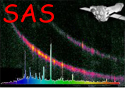XMM-Newton Science Analysis System

param (param-2.18) [xmmsas_20211130_0941-20.0.0]

## angle

Angle parameters are intended for right ascensions and declinations, where conversion from hours/minutes/seconds or degrees/minutes/seconds may be needed. For other angles, such as small offset angles, a real parameter is more appropriate. Note that the maximum range of the angle type is [-360,360] degrees.

The angle can be entered in one of the following formats:

```   (+|-)xxdxxmxx[.xxx]s      (degrees, minutes and seconds)
xxhxxmxx[.xxx]s           (hours, minutes and seconds)
[(+|-)]xxx[.xxx]          (decimal degrees)
```

where `x' is a single digit, `d',`m',`s' and `h` are literal characters and square brackets denote optional items. The number of digits shown is not significant.

Note: Angle parameters are accessed by the task as a real value, in degrees.

XMM-Newton SOC -- 2021-11-30